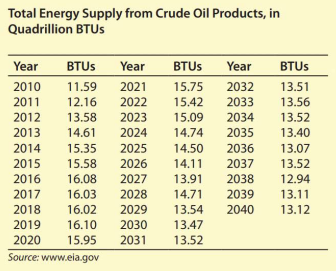# II. Energy from Crude Oil (Modeling) The table shows the total energy supply from crude oil products, in quadrillion BTUs, for the years from 2010 and projected through 2040. What can we conclude about the derivative on either side of the x -coordinate of the point ( 25.36 , 12.958 ) ?### Mathematical Applications for the ...

11th Edition
Ronald J. Harshbarger + 1 other
Publisher: Cengage Learning
ISBN: 9781305108042

#### Solutions

Chapter
Section### Mathematical Applications for the ...

11th Edition
Ronald J. Harshbarger + 1 other
Publisher: Cengage Learning
ISBN: 9781305108042
Chapter 9, Problem 10EAGP2
Textbook Problem
1 views

## II. Energy from Crude Oil (Modeling)The table shows the total energy supply from crude oil products, in quadrillion BTUs, for the years from 2010 and projected through 2040.What can we conclude about the derivative on either side of the x-coordinate of the point ( 25.36 ,   12.958 ) ?

This textbook solution is under construction.

### Still sussing out bartleby?

Check out a sample textbook solution.

See a sample solution

#### The Solution to Your Study Problems

Bartleby provides explanations to thousands of textbook problems written by our experts, many with advanced degrees!

Get Started